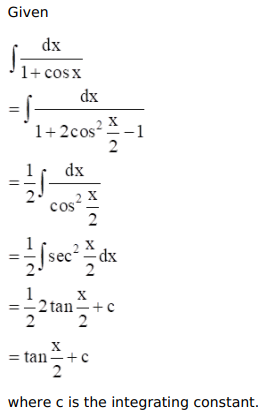# Mark against the correct answer in each of the following:

Question:

Mark $(\sqrt{)}$ against the correct answer in each of the following:

$\int \frac{d x}{(1+\cos x)}=?$

A. $\frac{1}{2} \tan \frac{x}{2}+C$

B. $-\cot \frac{x}{2}+C$

C. $\tan \frac{x}{2}+C$

D. none of these

Solution: